Skip to main content

# Solving the fractional nonlinear Klein-Gordon equation by means of the homotopy analysis method

## Abstract

In this paper, the homotopy analysis method is applied to obtain the solution of nonlinear fractional partial differential equations. The method has been successively provided for finding approximate analytical solutions of the fractional nonlinear Klein-Gordon equation. Different from all other analytic methods, it provides us with a simple way to adjust and control the convergence region of solution series by introducing an auxiliary parameter ħ. The analysis is accompanied by numerical examples. The algorithm described in this paper is expected to be further employed to solve similar nonlinear problems in fractional calculus.

## 1 Introduction

In this paper, we consider the fractional nonlinear Klein-Gordon equation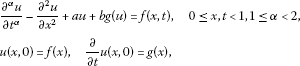where u is a function of x and t, a and b are real, g is a nonlinear function, and f is a known analytic function. The Klein-Gordon equation plays an important role in mathematical physics.

The homotopy perturbation method (HPM) has been successively applied for finding approximate analytical solutions of the fractional nonlinear Klein-Gordon equation which can be used as a numerical algorithm . Analytical approach that can be applied to solve nonlinear differential equations is to employ the homotopy analysis method (HAM) . Chowdhury and Hashim have employed HPM for solving Klein-Gordon equations . The main aim of this work is to apply the HPM to solve the nonlinear Klein-Gordon equations of fractional order. An account of the recent developments of HAM was given by Liao . HAM has been successfully applied in engineering fields. The method has been applied to give an explicit solution for the Riemann problem of the nonlinear shallow-water equations . The homotopy analysis method is applied to solve linear and nonlinear fractional partial differential equations (fPDEs) . The obtained Riemann solver has been implemented into a numerical model to simulate long waves, such as storm surge or tsunami, propagation and run-up. Differential equations and nonlinear mechanics very recently, Song and Zhang  solved the fractional KdV-Burgers-Kuramoto equation using HAM. Cang et al.  solved nonlinear Riccati differential equations of fractional order using HAM. Hashim et al.  employed HAM to solve fractional initial value problems (fIVPs) for ordinary differential equations. In  the applicability of HAM was extended to construct a numerical solution for the fractional BBM-Burgers equation. The homotopy analysis method is implemented to give approximate and analytical solutions for the Klein-Gordon equation . The HAM solutions for systems of nonlinear fractional differential equations were presented by Bataineh et al. . A specific linear, nonhomogeneous time fractional partial differential equation (fPDE) with variable coefficients was first transformed into two fractional ordinary differential equations, which were then solved by HAM in . Recently, Xu et al.  applied HAM to linear, homogeneous one- and two-dimensional fractional heat-like PDEs subject to the Neumann boundary conditions. They implemented relatively new, exact series method of solution known as the differential transform method for solving linear and nonlinear Klein-Gordon equations . Jafari and Seifi  applied HAM to linear and nonlinear homogeneous fractional diffusion-wave equations. Very recently, HAM was shown to be capable of solving linear and nonlinear systems of fPDEs .

## 2 Definitions

### 2.1 The Mittag-Leffler function

The Mittag-Leffler function is an important function that finds widespread use in the world of fractional calculus. Just as the exponential naturally arises out of the solution to integer order differential equations, the Mittag-Leffler function plays an analogous role in the solution of non-integer order differential equations. In fact, the exponential function itself is a very special form, one of an infinite set, of this seemingly ubiquitous function. Here, m th derivatives of Mittag-Leffler functions  are given

$E α ( m ) (z)= ∑ k = 0 ∞ ( k + m ) ! z k k ! Γ ( α k + α m + 1 ) ,z,α∈C,m∈N,R(α)>0.$

A two-parameter function of the M-L (Mittag-Leffler) type is defined by the series expansion ,

$E α ( m ) (z)= ∑ k = 0 ∞ ( k + m ) ! z k k ! Γ ( α k + α m + β ) ,α,β∈C,R(α)>0,R(β)>0,z∈C,m∈N.$

### 2.2 Laplace’s transform of fractional order

The Laplace transform of a function $f(t)$, denoted by $F(s)$, is defined by the equation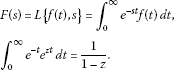If $n∈N$, then by the theory of the Laplace transform, we know that

$L { d d x n ; f ; s } = s n F(s)− ∑ k = 0 n − 1 s n − k − 1 f ( k ) (0+)$

or

$L { D α ; f ; s } = s α F(s)− ∑ k = 0 n − 1 s α − k − 1 f ( k ) (0+)(n−1≤α

In this section several integrals associated with Mittag-Leffler functions are presented, which can be easily established by the application of beta and gamma function formulas and other techniques ,

$∫ 0 ∞ e − t t k e z t dt= ( k + 1 ) ! ( 1 − z ) k and ∫ 0 ∞ e − p t t k e a t dt= k ! ( p − a ) k + 1 ( Re ( p ) > a ) .$

We obtain, from the equation, a pair of Laplace transforms of the function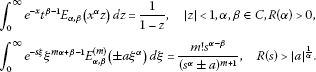### 2.3 Fractional calculus

We have well-known definitions of a fractional derivative of order $α>0$ such as Riemann-Liouville, Grunwald-Letnikow, Caputo, and generalized functions approach [23, 24]. The most commonly used definitions are those of Riemann-Liouville and Caputo. We give some basic definitions and properties of the fractional calculus theory, which are used throughout the paper.

Definition 2.1 A real function $f(x)$, $x>0$, is said to be in the space $C μ$, $μ∈R$, if there exists a real number ($p>μ$) such that $f(x)= x p f 1 (x)$, where $f 1 (x)∈C[0,∞)$, and it is said to be in the space $C μ m$ iff $f m ∈ C μ$, $m∈N$.

Definition 2.2 The Riemann-Liouville fractional integral operator of order $α≥0$ of a function $f∈ C μ$, $μ≥−1$, is defined as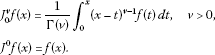It has the following properties. For $f∈ C μ$, $μ≥−1$, $α,β≥0$, and $γ>1$,

1. 1.

$J α J β f(x)= J α + β f(x)$,

2. 2.

$J α J β f(x)= J β J α f(x)$,

3. 3.

$J α x γ = Γ ( γ + 1 ) Γ ( α + γ + 1 ) x α + γ$.

The Riemann-Liouville fractional derivative is mostly used by mathematicians, but this approach is not suitable for physical problems of the real world since it requires the definition of fractional order initial conditions which have no physically meaningful explanation yet. Caputo introduced an alternative definition which has the advantage of defining integer order initial conditions for fractional order differential equations.

Definition 2.3 The fractional derivative of $f(x)$ in the Caputo sense is defined as

$D ∗ v f(x)= J a m − v D m f(x)= 1 Γ ( m − v ) ∫ 0 x ( x − t ) m − v − 1 f ( m ) (t)dt$

for $m−1, $m∈N$, $x>0$, $f∈ C − 1 m$.

Lemma 2.4 If $m−1<α, $m∈N$, and $f∈ C μ m$, $μ≥−1$, then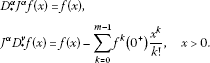The Caputo fractional derivative is used here because it allows traditional initial and boundary conditions to be included in the formulation of the problem.

Definition 2.5 For m to be the smallest integer that exceeds α, the Caputo time-fractional derivative operator of order $α>0$ is defined as

## 3 Homotopy analysis method

We apply the homotopy analysis method to the discussed problem. Let us consider the fractional differential equation,

$FD ( u ( x , t ) ) =0.$

Based on the constructed zero-order deformation equation by Liao (2003), we give the following zero-order deformation equation in the similar way:

$(1−q)L ( U ( x , t ; q ) − u 0 ( x , t ) ) =qħFD ( U ( x , t ; q ) ) ,q∈[0,1],ħ≠0,$

L is an auxiliary linear integer order operator and it possesses the property $L(C)=0$, U is an unknown function.

Expanding U in Taylor series with respect to q, one has

$U(x,t;q)= u 0 (x,t)+ ∑ m = 1 ∞ u m (x,t) q m ,$

where

$u m (x,t)= ∂ U ( x , t ; q ) ∂ q m | q = 0 .$

Differentiating the equation m times with respect to the embedding parameter q, then setting $q=0$, and finally dividing them by m!, we have the m th-order deformation equation

$L [ u m ( x , t ) − χ m u m − 1 ( x , t ) ] =ħ R m [ u → m − 1 ( x , t ) ] ,$

where

$R m [ u → m − 1 ( x , t ) ] = 1 ( m − 1 ) ! ∂ m − 1 F D ( U ( x , t ; q ) ) ∂ q m − 1 | q = 0$

and

$χ m ={ 0 , m ≤ 1 , 1 , m > 1 .$

These equations can be easily solved using software such as Maple, Mathlab and so on.

## 4 Application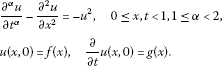We rewrite the equation in an operator form,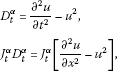which gives, according to Caputo, that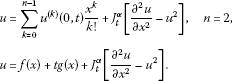We define, according to the equation, the linear and nonlinear operator,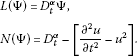According to the equation (Klein-Gordon equation), using the homotopy analysis method,

$J t α D t α [ u m ( x , t ) − x m − 1 u m − 1 ( x , t ) ] =ħ J t α { R m [ u → m − 1 ( x , t ) ] } ,$

where

$R m [ u → m − 1 ( x , t ) ] = D t α u m − 1 − [ ∂ 2 u ∂ x 2 − ∑ j = 0 m − 1 u j u m − 1 − j ] ,$

we find

$u 1 =−ħ J t α [ ∂ 2 u 0 ∂ x 2 − u 0 2 ] .$

Now, the solution of the m th-order deformation equation for $m≥1$ becomes

$u m =(ħ+1) u m − 1 (x,t)−ħ J t α [ ∂ 2 u ∂ x 2 − ∑ j = 0 m − 1 u j u m − 1 − j ] .$

Consider the fractional nonlinear partial differential equation: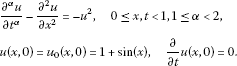We now successively obtain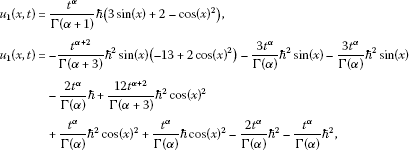so

$u(x,0)≅ u 0 (x,0)+ u 1 (x,0)+ u 2 (x,0).$

For the special case $h=−1$, we obtain from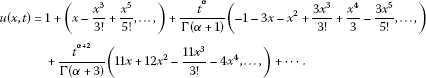We have given the solution simulations in Figure 1 and Figure 2 according to different α values.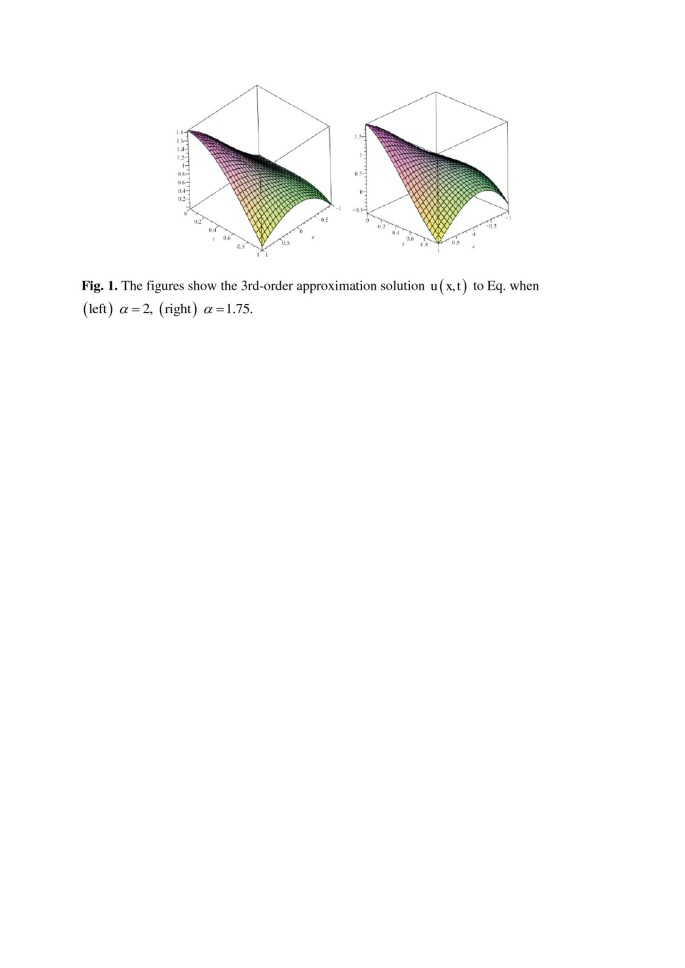Figure 1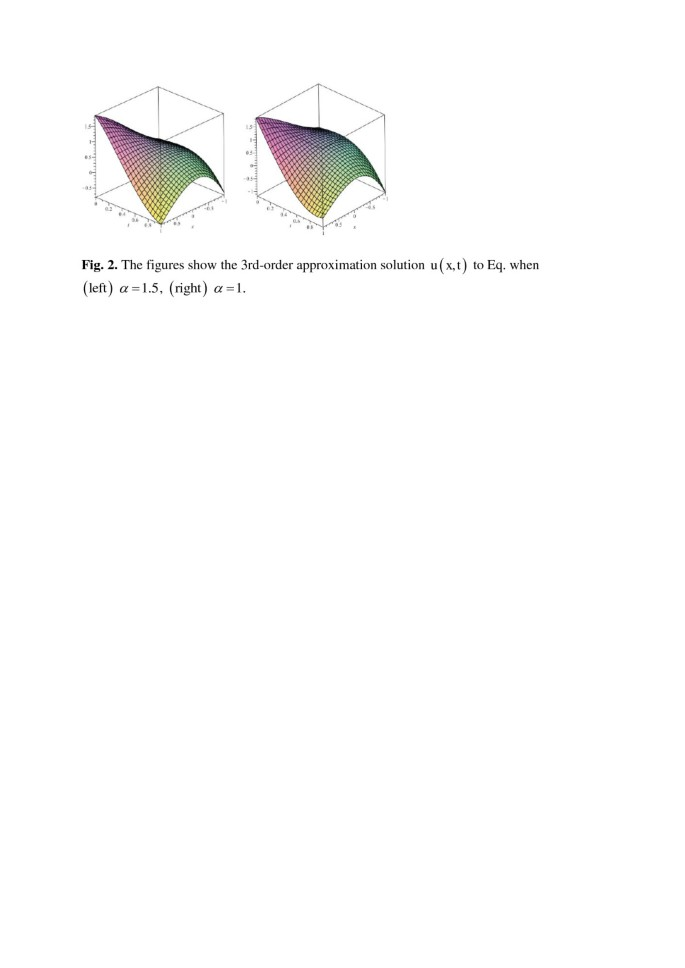Figure 2

## 5 Conclusion

In this study, the homotopy analysis method with new strategies has been employed to obtain an approximate analytical solution of fractional nonlinear Klein-Gordon equations. It is quite important to notice that a higher number of iteration and higher order of p are needed to gain more accuracy.

This work illustrates the validity and great potential of the homotopy analysis method for nonlinear fractional partial differential equations. The basic ideas of this approach are expected to be further employed to solve other nonlinear problems in fractional calculus.

## References

1. 1.

Golmankhaneh AK, Golmankhaneh AK, Baleanu D: On nonlinear fractional Klein-Gordon equation. Signal Process. 2011, 91: 446–451. 10.1016/j.sigpro.2010.04.016

2. 2.

Liao, S-J: The proposed homotopy analysis technique for the solution of nonlinear problems. PhD thesis, Shanghai Jiao Tong University, Shanghai, China (1992)

3. 3.

Liao S-J: An approximate solution technique not depending on small parameters: a special example. Int. J. Non-Linear Mech. 1995, 30(3):371–380. 10.1016/0020-7462(94)00054-E

4. 4.

Liao S-J: A kind of approximate solution technique which does not depend upon small parameters - II. An application in fluid mechanics. Int. J. Non-Linear Mech. 1997, 32(5):815–822. 10.1016/S0020-7462(96)00101-1

5. 5.

Liao S-J CRC Series: Modern Mechanics and Mathematics 2. In Beyond Perturbation: Introduction to the Homotopy Analysis Method. Chapman & Hall/CRC, Boca Raton; 2004.

6. 6.

Chowdhury MSH, Hashim I: Application of homotopy-perturbation method to Klein-Gordon and sine-Gordon equations. Chaos Solitons Fractals 2009, 39: 1928. 10.1016/j.chaos.2007.06.091

7. 7.

Liao S-J: Notes on the homotopy analysis method: some definitions and theorems. Commun. Nonlinear Sci. Numer. Simul. 2009, 14(4):983–997. 10.1016/j.cnsns.2008.04.013

8. 8.

Wu Y, Cheung KF: Explicit solution to the exact Riemann problem and application in nonlinear shallow-water equations. Int. J. Numer. Methods Fluids 2008, 57(11):1649–1668. 10.1002/fld.1696

9. 9.

Abdulaziz O, Hashim I, Saif A: Series solutions of time-fractional PDEs by homotopy analysis method. Differ. Equ. Nonlinear Mech. 2008., 2008: Article ID 686512. doi:10.1155/2008/686512

10. 10.

Song L, Zhang H: Application of homotopy analysis method to fractional KdV-Burgers-Kuramoto equation. Phys. Lett. A 2007, 367(1–2):88–94. 10.1016/j.physleta.2007.02.083

11. 11.

Cang J, Tan Y, Xu H, Liao S-J: Series solutions of non-linear Riccati differential equations with fractional order. Chaos Solitons Fractals 2009, 40: 1–9. 10.1016/j.chaos.2007.04.018

12. 12.

Hashim I, Abdulaziz O, Momani S: Homotopy analysis method for fractional IVPs. Commun. Nonlinear Sci. Numer. Simul. 2009, 14(3):674–684. 10.1016/j.cnsns.2007.09.014

13. 13.

Song L, Zhang H: Solving the fractional BBM-Burgers equation using the homotopy analysis method. Chaos Solitons Fractals 2009, 40: 1616–1622. 10.1016/j.chaos.2007.09.042

14. 14.

Alomari AK, Noorani MSM, Nazar RM: Approximate analytical solutions of the Klein-Gordon equation by means of the homotopy analysis method. JQMA 2008, 4(1):45–57.

15. 15.

Bataineh AS, Alomari AK, Noorani MSM, Hashim I, Nazar R: Series solutions of systems of nonlinear fractional differential equations. Acta Appl. Math. 2009, 105(2):189–198. 10.1007/s10440-008-9271-x

16. 16.

Xu H, Cang J: Analysis of a time fractional wave-like equation with the homotopy analysis method. Phys. Lett. A 2008, 372(8):1250–1255. 10.1016/j.physleta.2007.09.039

17. 17.

Xu H, Liao S-J, You X-C: Analysis of nonlinear fractional partial differential equations with the homotopy analysis method. Commun. Nonlinear Sci. Numer. Simul. 2009, 14(4):1152–1156. 10.1016/j.cnsns.2008.04.008

18. 18.

Ravi Kanth ASV, Aruna K: Differential transform method for solving the linear and nonlinear Klein-Gordon equation. Comput. Phys. Commun. 2009, 180: 708–711. 10.1016/j.cpc.2008.11.012

19. 19.

Jafari H, Seifi S: Homotopy analysis method for solving linear and nonlinear fractional diffusion-wave equation. Commun. Nonlinear Sci. Numer. Simul. 2009, 14(5):2006–2012. 10.1016/j.cnsns.2008.05.008

20. 20.

Jafari H, Seifi S: Solving a system of nonlinear fractional partial differential equations using homotopy analysis method. Commun. Nonlinear Sci. Numer. Simul. 2009, 14(5):1962–1969. 10.1016/j.cnsns.2008.06.019

21. 21.

Mittag-Leffler G:Sur la nouvelle fonction $E α (x)$. Comptes Rendus Hebdomadaires Des Seances Del Academie Des Sciences, Paris 2 1903, 137: 554–558.

22. 22.

Wiman A: Über den fundamental Satz in der Theorie der Funktionen. Acta Math. 1905, 29: 191–201. 10.1007/BF02403202

23. 23.

Podlubny I: Fractional Differential Equations. Academic Press, San Diego; 1999.

24. 24.

Caputo M: Geophys. J. R. Astron. Soc.. 1967, 13: 529–539. 10.1111/j.1365-246X.1967.tb02303.x

Download references

## Acknowledgements

This work was supported by the scientific and technological research council of Turkey (TUBITAK).

## Author information

Authors

### Corresponding author

Correspondence to Muhammet Kurulay.

## Additional information

### Competing interests

The author declares that he has no competing interests.

## Authors’ original submitted files for images

Below are the links to the authors’ original submitted files for images.

## Rights and permissions

Open Access This article is distributed under the terms of the Creative Commons Attribution 2.0 International License (https://creativecommons.org/licenses/by/2.0), which permits unrestricted use, distribution, and reproduction in any medium, provided the original work is properly cited.

Reprints and Permissions

## About this article

### Cite this article

Kurulay, M. Solving the fractional nonlinear Klein-Gordon equation by means of the homotopy analysis method. Adv Differ Equ 2012, 187 (2012). https://doi.org/10.1186/1687-1847-2012-187

Download citation

• Received:

• Accepted:

• Published:

### Keywords

• fractional nonlinear Klein-Gordon equations
• homotopy analysis method
• analytical solutions